Follow

# A Basic Understanding of Surfer Gridding Methods – Part 1

Surfer offers many different grid based maps, such as 2D contour maps and 3D surface maps. To create a grid based map, you must have a grid file. To create a grid file, Surfer takes randomly spaced XYZ data and uses it to create a regularly spaced grid file, composed of grid nodes. Each grid node is located at a particular XY location and has a Z value associated with it. Although the algorithms are computed internally in Surfer, choosing the best gridding method for the data can be difficult. Surfer has several different options for gridding methods and each have its own set of gridding options. This article focuses on six of the gridding methods and a basic understanding of each; the rest of the gridding methods are covered in A Basic Understanding of Surfer Gridding Methods – Part 2. The methods that are examined are Kriging, Nearest Neighbor, Natural Neighbor, Local Polynomial, Radial Basis Function, and Triangulation with Linear Interpolation.

### Kriging

Kriging is one of the more flexible and accurate gridding methods; typically the one that is recommended when gridding data. Kriging is effective because it produces a good map for most data sets. It also can compensate for clustered data by giving less weight to the cluster in the overall prediction. One of the disadvantages to Kriging is that it can be slower than other methods. It also can extrapolate grid values beyond the range of the data’s Z values. Surfer has some different options of Kriging available to customize.

Each grid node value is based on the known data points neighboring the node. Each data point is weighted by its distance away from the node. This way, points that are further from the node will have less weight in the estimation of the node. For example, to compute the Z value at grid node A, this equation is used:Where ZA is the estimated value of grid node A, n is the number of neighboring data values used in the estimation, Zi is the value at location i with weight, Wi. The value of weights will sum to 1 to make sure there is no bias towards clustered data points. The formula can get more complex if things such as drifts and a search radius are applied.

Below is a classed post map displaying a set of data values that were gridded using the Kriging method.The classed post map, left, represents scattered elevation data that produced the contour map, right, using the Kriging gridding method.

In Surfer, default properties of Kriging are point Kriging with no drifts and a circular search ellipse. The default properties are used unless otherwise specified. To set the gridding method to Kriging, in the Grid Data dialog, change the Gridding Method to Kriging. To customize Kriging, click on Advanced Options to bring up the Grid Data Advanced Options.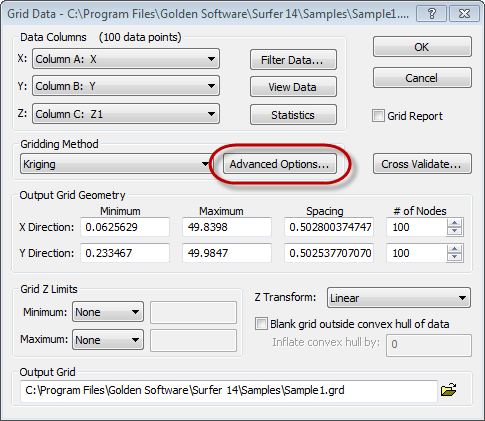Click the Advanced Options button to open the Grid Data Advanced Options dialog.

The Grid Data Advanced Options dialog has different sections that contain different specialization sections. The Kriging Parameters section, shown below, has four settings: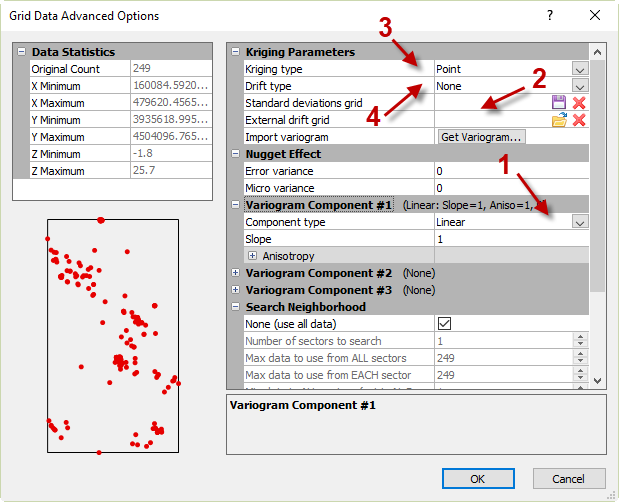The dialog contains customizable options to best fit the Kriging gridding method to the data.
1. Variogram Model:
A variogram is a description of the surface’s roughness based quantitatively and statistically. The variogram is the degree of variance between values at two locations, x and y. This section is useful for adding components to the variogram produced with the Kriging gridding method. For example, a nugget can be added to the variogram, which represents a jump at the origin for the semivariogram. If you are not sure what components to add, it is best to use the default Linear variogram, with no nugget effect. For more information on variograms, download this PDF tutorial.
2. Output Grid of Kriging Standard Deviations:
You can specify a standard deviation estimation grid to be created. The Z value in the estimation grid is the difference between the actual grid node value and the expected value. Choosing to have a standard deviation grid calculated will increase the amount of time it takes to grid your data. The standard deviation grid is useful when:
1. The variogram model is representative of the data.
2. The standard deviation grids are correctly scaled due to using the Standardized Variogram estimator or the Autocorrelation estimator.
3. The default linear model does not have a slope of one.
4. The No Search option is not selected on the Search tab.
3. Kriging Type:
There are two types of Kriging available in Surfer: point Kriging and block Kriging. Point Kriging, the default, is simple and estimates the value of a point from a set of nearby values. Block Kriging estimates the average value of the rectangular blocks centered on the grid nodes. The blocks are the size and shape of a grid cell. The advantage to use Block Kriging is that it often provides better variance estimates and smooth interpolated results. However, Block Kriging is not an exact interpolator because it is not estimating the value at a point. If the neighboring cells share a data point then that point is used to estimate the value of the grid node in each of the blocks.
4. Drift Type:
Choosing the default, no drift, will keep it as ordinary Kriging. However, choosing a linear or quadratic drift will change it to universal kriging. Ordinary Kriging is a linear prediction assuming a stationary constant, unknown mean. This means that the data is more predictable and can be forecasted, such as economic data that reflects a seasonal trend. On the contrary, Universal Kriging is a linear prediction assuming a nonstationary mean structure. This means that the data is unpredictable and hard to forecast, such as wind speed.

The Search tab allows you to specify the search options of which points are considered when interpolating grid nodes.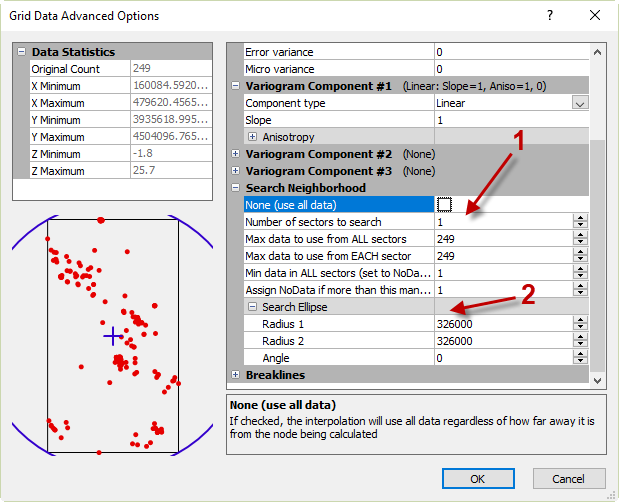The Search Neighborhood section contains setting to modify the search parameters of the selected gridding method.
1. Search options: The options set in the Search tab allow Surfer to know which data points to use when interpolating the grid nodes. It can be useful to specify the search settings for different types of data sets, such as different sizes of data sets. For example, if the data set is small (under 250 data points) then it is more reasonable to use the No Search option and interpolate the grid nodes with all of the data. On the contrary, if the data set is large then it may be ideal to limit the number of data points used to interpolate each grid node.
1. No Search: When this option is selected then Surfer will use all of the data points in estimating the grid file. This is useful for smaller data sets, up to 250 points. This is also adequate for evenly distributed data. If this option is disabled, it is because there are more than 750 data points and Surfer cannot grid the data without breaking the file into smaller sectors.
2. Number of sectors to search: Allows the search area to be divided into sections, up to 32 search sectors can be specified. This is ideal for data that is clustered.
3. Maximum number of data to use for ALL sectors: This limits the total number of points used in the overall estimation.
4. Maximum number of data to use from EACH sector: This value dictates the number of points to be used from each sector.
5. Minimum number of data in all sectors (node is set to NoData if fewer): If there are not enough points found when estimating a grid node then Surfer will assign that grid node the NoData value. The grid nodes with NoData values represent insufficient data and will trim the contour lines on the map.
6. Assign NoData to node if more than this many sectors are empty: If there are not enough sectors for the grid node then Surfer will assign that grid node the NoData value.
2. Search Ellipse: The search ellipse specifies the size of the local neighborhood in which to look for data, this can be impacted by the search rules set. The values of radius 1 and radius 2 are the distance in positive data units. The radii values are the lengths that are searched in the direction indicated by the angle. The angle is represented in orientation between the positive x axis and the radius 1 of the ellipse axis. If there are no points found in the search ellipse then that grid node will be assigned the NoData value. By default, the search ellipse is circular giving equal weight to both directions surrounding the grid node. The default length is half the diagonal distance of the data.

The search options can be customized so that large areas that have no data points are assigned the NoData value. To do this, set the radius of the search ellipse equal to a value that is less than the distance between data values.

The Breaklines tab allows you to enter in a boundary file with the line vertices defined with X, Y, and Z values.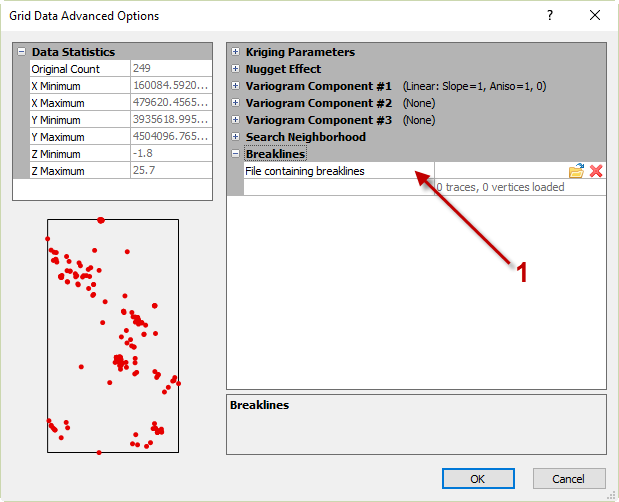The Breaklines section is used to upload a blanking file with the breaklines information.
1. Breaklines: Kriging supports breaklines but not faults. A breakline is an interruption in a surface’s slope that is often wavy and irregular, such as the base of a cliff. These are useful to represent any irregularities and disconformities in the map. Breaklines are not barriers when gridding; this means that all data points on both sides of the line are used when interpolating the grid nodes, regardless of the position on either side of the line. However, if a grid node is located on the boundary line then the value of the grid node becomes the value of the boundary file.

The breakline file contains the X, Y, and Z vertices of the interruption in a blanking file (*.BLN) format: the first row is the number of data points in the file followed by the XYZ coordinates of the line.

### Nearest Neighbor

Nearest Neighbor is best used with regularly spaced data points. If the observations have a few missing data points, this method is best for filling in the holes. This method is an exact interpolator and does not extrapolate beyond the Z range of the data.

The Nearest Neighbor method is not mathematically intense. When using Nearest Neighbor, the Z value of each grid node is simply the Z value of the nearest original data point to that grid node. The nearest neighbor to a grid node uses a simple separation distance without taking anisotropy into account. If two or more points tie as the nearest neighbor, the tied data points are sorted on X, then Y, and then Z values. The smallest value is selected as the nearest neighbor.

The example below shows a data set that was gridded using the Nearest neighbor gridding method.The classed post map, left, was created with the data points. The contour map, left, represents the interpolated grid nodes using the Nearest Neighbor gridding method.

The Nearest Neighbor is naturally simple; however, you can specify the search radius and add a breakline/fault to the algorithm. To specify the advanced options of the Nearest Neighbor gridding method, in the Grid Data dialog, click Advanced Options.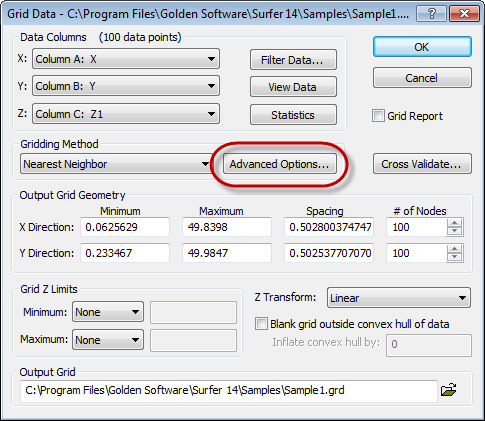Click the Advanced Options button to open the Grid Data Advanced Options dialog.

The Search section allows you to specify the radii and angles of the search ellipse.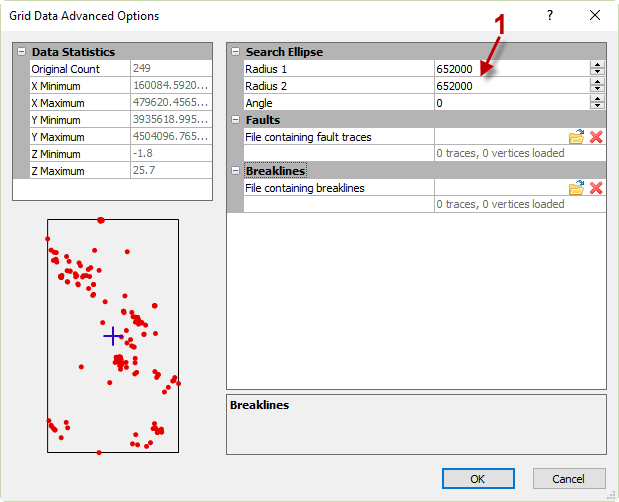The Search section contains the settings for the search ellipse.
1. The search ellipse specifies the size of the local neighborhood in which to look for data, this can be impacted by the search rules set. The values of radius 1 and radius 2 are the distance in positive data units. The radii values are the lengths that are searched in the direction indicated by the angle. The angle is represented in orientation between the positive x axis and the radius 1 of the ellipse axis. If there are no points found in the search ellipse then that grid node will be assigned the NoData value. By default, the search ellipse is circular giving equal weight to both directions surrounding the grid node. The default length is the diagonal distance of the data.

The search options can be customized so that large areas that have no data points are assigned the NoData value. To do this, set the radius of the search ellipse equal to a value that is less than the distance between data values.

The Breaklines and Faults section allows for you to specify the boundary files for the breaklines and faults to be used in gridding.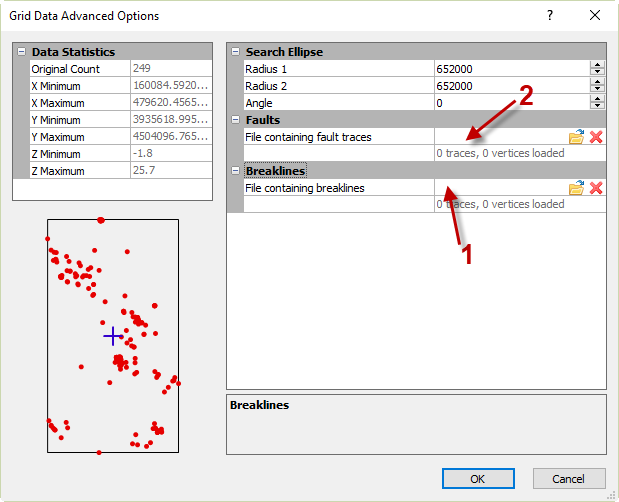The Breaklines and Faults section is used to upload a blanking file with the breaklines and faults information.
1. A breakline is an interruption in a surface’s slope that is often wavy and irregular, such as the base of a cliff. These are useful to represent any irregularities and disconformities in the map. Breaklines are not barriers when gridding; this means that all data points on both sides of the line are used when interpolating the grid nodes, regardless of the position on either side of the line. However, if a grid node is located on the boundary line then the value of the grid node becomes the value of the boundary file.

The breakline file contains the X, Y, and Z vertices of the interruption in a blanking file (*.BLN) format: the first row is the number of data points in the file followed by the XYZ coordinates of the line.
2. A fault is a 2D line that acts as a barrier when gridding data. The fault lines represent a significant discontinuity along fractures due to the movement of the earth. In gridding, the fault line acts as a barrier of information when gridding. The data on one set of the fault is not used when interpolating the values on the other side of the fault line.

A fault file contains the X and Y values of the line that represent the fault in a blanking file (*.BLN) format: the first row is the number of data points in the file followed by the XY coordinates of the line.

### Natural Neighbor

The natural neighbor gridding method is popular with data sets that have dense data in some areas and sparse data in other areas. The Natural Neighbor estimates the grid node value by finding the closest subset of input data points to a grid node and then applying weight to each. The Natural Neighbor method does not extrapolate the Z grid values beyond the range of data and it does not generate nodes in areas without data.

Below is an example of evenly spaced data and the grid file that is produced when using Natural Neighbor as the gridding method.The classed post map, left, was created with the data points. The contour map, left, represents the interpolated grid nodes using the Natural Neighbor gridding method.

The Natural Neighbor method has options to set the anisotropy and to save the Delaunay triangles. To specify the advanced option of the Natural Neighbor gridding method, in the Grid Data dialog, click Advanced Options.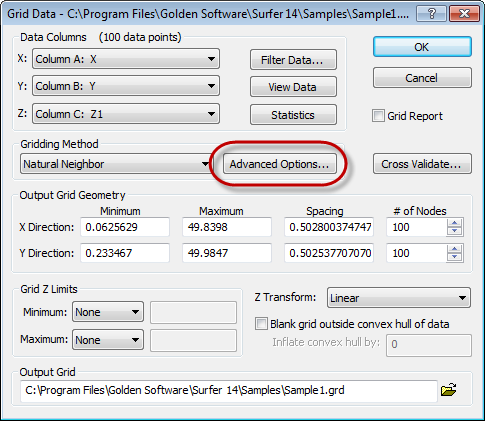Click the Advanced Options button to open the Grid Data Advanced Options dialog.

Anisotropy is useful in eliminating trends in the data when interpolating the grid nodes. Anisotropy refers to directionally dependent data. The Grid Data Advanced Options dialog allows you to specify three different customizations of the gridding. The customizable areas: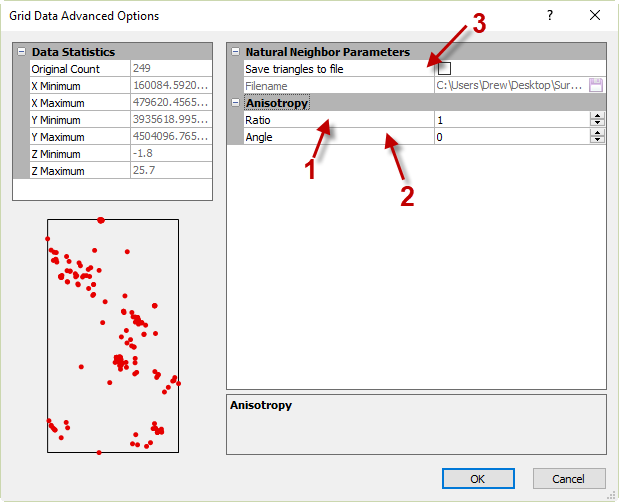The Grid Data Advanced Options allows the anisotropy settings to be specified when gridding the data.
1. Anisotropy Ratio: This value defines the relative weight of the points when interpolating the grid nodes. This value is computed by dividing the maximum range by the minimum range. For most data sets, it is recommended to accept the default value of 1, which means that all points have the same weight. You may want to increase the ratio of the points in one direction that has more similarity than the points in another direction. By increasing the ratio, the points in the direction defined will have more weight on the value of the interpolated grid node. The ratio is typically considered severe if greater than four and mild if less than two.
2. Anisotropy Angle: The angle is the direction of the major axis, in degrees. The angle rotates counterclockwise with 0 degrees being east-west and 90 degrees being north-south going multiple directions. In order to use a direction angle, an anisotropy angle should not equal 1.
3. Save Triangles To: The Delaunay triangles are formed by connecting lines between data points. Often the triangles are formed by maximizing the minimum angle; this prevents small triangles from being formed. The result is triangular faces all over the grid. If two or points are located on the same line then there is no Delaunay triangulation. The triangles that are formed are not intersected by other triangles. The triangles can be useful to determine the density of the data points. The Delaunay triangles can be loaded as a base map and combined with other maps. To save the file, click the folder icon and specify the location and file format to save the file.

### Local Polynomial

Local polynomial interpolation works best with data sets that are relatively smooth within search neighborhoods. This method fits an ordered polynomial using points within a defined neighborhood. The different polynomials allows for different fits through the data. For example, a single-order polynomial fits a plane through the data; a second-order fits a surface with a bend; a third-order accommodates two bends, and so on. If the surface being gridded has multiple shapes, such as repetitive sloping and leveling then it is best to use multiple polynomial planes. The multiple polynomial planes would represent the surface more accurately. This method is useful with larger data sets because the computation speed is not affected by the data set size.

A polynomial is fit against the data using weighted least squares. The grid nodes are assigned the value of the polynomial at each node. Surfer allows the polynomials to be of order 1, 2, or 3. The data that is closer to the grid node has a higher weight than the data that is further away.

The example below shows a data set that was gridded using the Local Polynomial gridding method.The classed post map, left, was created with the data points. The contour map, left, represents the interpolated grid nodes using the Local Polynomial gridding method.

To specify the advanced options of the Local Polynomial gridding method, in the Grid Data dialog, click Advanced Options.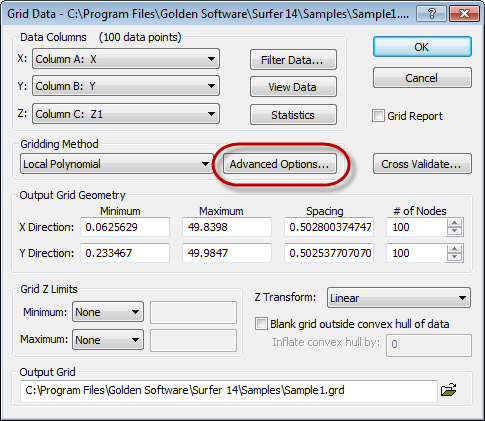Click the Advanced Options button to open theGrid Data Advanced Options dialog.

The Local Polynomial Parameters section of the Grid Data Advanced Options displays the Power and Polynomial Order properties.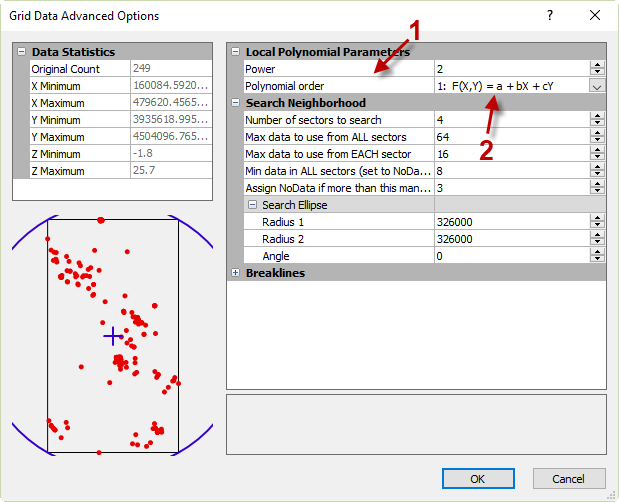The General section in the Grid Data Advanced Options dialog is used to customize the power and polynomial order of the gridding method.
1. Power: The power specified is applied to the weight calculation that is used to minimize the sum of the squared residuals. Set the power to a value between 0 and 20.
2. Polynomial Order: This allows you to select the order of the polynomial that you would like to use to interpolate the data.

The Search Neighborhood section allows you to specify the search options of which points are considered when interpolating grid nodes.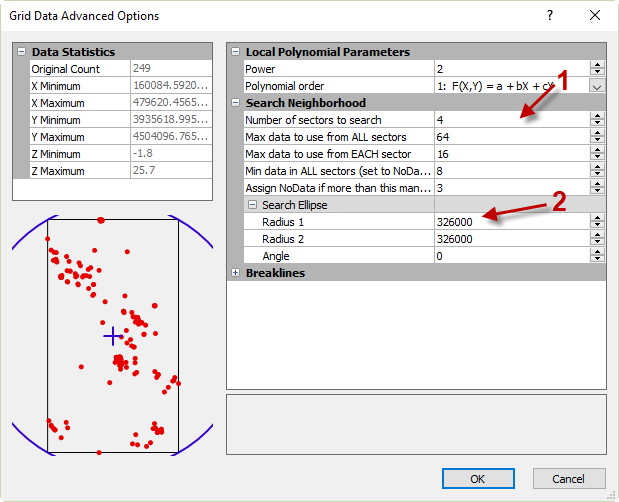The Search Neighborhood section contains setting to modify the search parameters of the selected gridding method.
1. Search options: The options set in the Search Neighborhood allow Surfer to know which data points to use when interpolating the grid nodes. It can be useful to specify the search settings for different types of data sets, such as different sizes of data sets.
1. Number of sectors to search: Allows the search area to be divided into sections, up to 32 search sectors can be specified. This is ideal for data that is clustered.
2. Maximum number of data to use for ALL sectors: This limits the total number of points used in the overall estimation.
3. Maximum number of data to use from EACH sector: This value dictates the number of points to be used from each sector.
4. Minimum number of data in all sectors (node is set to NoData if fewer): If there are not enough points found when estimating a grid node then Surfer will assign that grid node the NoData value. The grid nodes with NoData values represent insufficient data and will trim the contour lines on the map.
5. Assign NoData to node if more than this many sectors are empty: If there are not enough sectors for the grid node then Surfer will assign that grid node the NoData value.
2. Search Ellipse: The search ellipse specifies the size of the local neighborhood in which to look for data, this can be impacted by the search rules set. The values of radius 1 and radius 2 are the distance in positive data units. The radii values are the lengths that are searched in the direction indicated by the angle. The angle is represented in orientation between the positive x axis and the radius 1 of the ellipse axis. If there are no points found in the search ellipse then that grid node will be assigned the NoData value. By default, the search ellipse is circular giving equal weight to both directions surrounding the grid node. The default length is half the diagonal distance of the data.

The search options can be customized so that large areas that have no data points are assigned the NoData value. To do this, set the radius of the search ellipse equal to a value that is less than the distance between data values.

The Breaklines section allows for you to specify the boundary files for the breaklines to be used in gridding.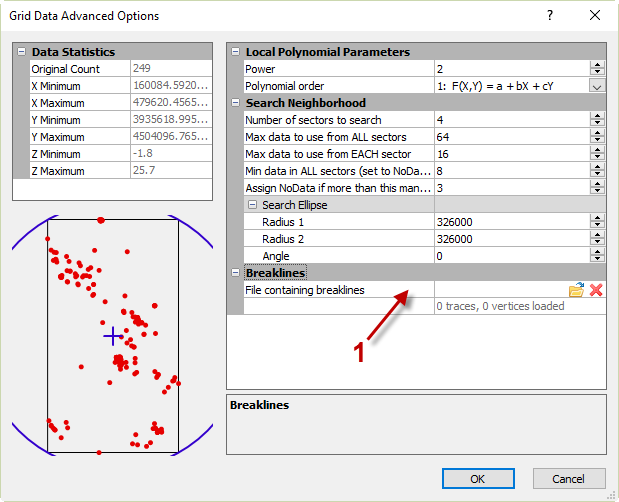The Breaklines section is used to upload a blanking file with the breaklines information.
1. A breakline is an interruption in a surface’s slope that is often wavy and irregular, such as the base of a cliff. These are useful to represent any irregularities and disconformities in the map. Breaklines are not barriers when gridding; this means that all data points on both sides of the line are used when interpolating the grid nodes, regardless of the position on either side of the line. However, if a grid node is located on the boundary line then the value of the grid node becomes the value of the boundary file.

The breakline file contains the X, Y, and Z vertices of the interruption in a blanking file (*.BLN) format: the first row is the number of data points in the file followed by the XYZ coordinates of the line.

### Radial Basis Function

The Radial Basis Function gridding method is an exact interpolator which means your data is honored. The real-value function depends on the distance from the origin. This method is similar to Kriging in that it is flexible and it generates an accurate interpretation of most data sets. The method can handle large sets of data and produce a smooth surface. However this is not an ideal method if the data has large changes in surface values within short distances. The Radial Basis Function method takes the user-specified function and fits it through the data values.

The example below shows a data set that was gridded using the Radial Basis Function gridding method.The classed post map, left, was created with the data points. The contour map, left, represents the interpolated grid nodes using the Radial Basis Function gridding method.

To specify the advanced options of the Radial Basis Function gridding method, in the Grid Data dialog, click Advanced Options.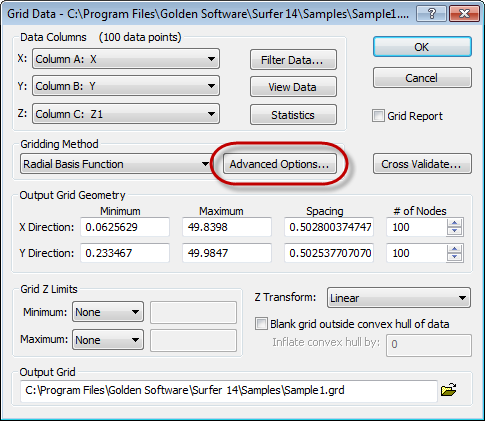Click the Advanced Options button to open theGrid Data Advanced Options dialog.

The Radial Basis Parameters section allows you to specify the Basis Function and R2 Parameter.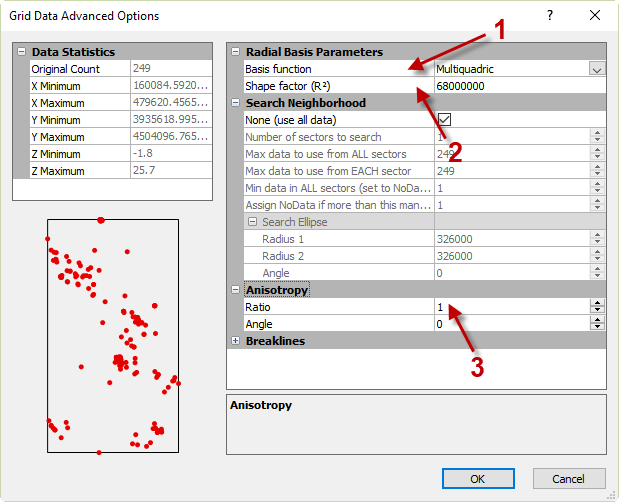The Radial Basis Parameters section contains some of the customizable settings for the gridding method.
1. Basis Function: Allows you to specify the function basis. The function chosen defines the optimal set of weights to apply to the data points when interpolating a grid node. The types of functions have the following equations:The functions above represent the functions that can be selected as the Basis Function for the gridding method.
2. R2 Parameter: R2 is referred to as the shaping factor. A larger R2 value will produce a map with smooth contour lines and round mountain tops. The value is calculated using this formula:
(length of diagonal of the data extent)2 / (25 * number of data points)
The Radial Basis Function is an exact interpolator, no matter what value is entered for R2.
3. Anisotropy Ratio: This value defines the relative weight of the points when interpolating the grid nodes. This value is computed by dividing the maximum range by the minimum range. For most data sets, it is recommended to accept the default value of 1, which means that all points have the same weight. You may want to increase the ratio of the points in one direction that has more similarity than the points in another direction. By increasing the ratio, the points in the direction defined will have more weight on the value of the interpolated grid node. The ratio is typically considered severe if greater than four and mild if less than two.

Anisotropy Angle: The angle is the direction of the major axis, in degrees. The angle rotates counterclockwise with 0 degrees being east-west and 90 degrees being north-south going multiple directions. In order to use a direction angle, an anisotropy angle should not equal 1.

The Search Neighborhood section allows you to specify the search options of which points are considered when interpolating grid nodes.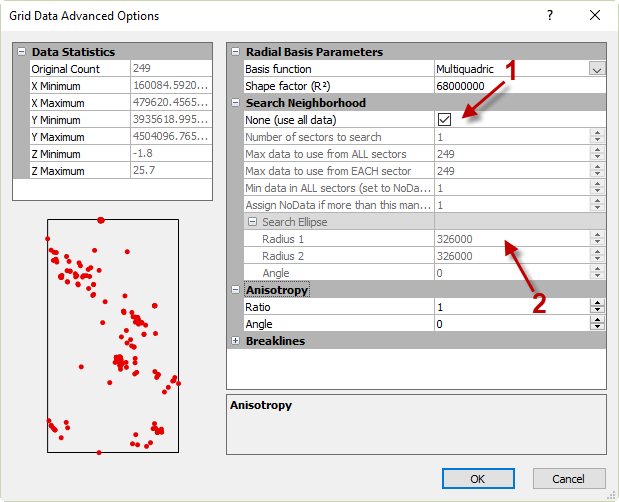The Search Neighborhood section contains setting to modify the search parameters of the selected gridding method.
1. Search options: The options set in the Search tab allow Surfer to know which data points to use when interpolating the grid nodes. It can be useful to specify the search settings for different types of data sets, such as different sizes of data sets. For example, if the data set is small (under 250 data points) then it is more reasonable to use the No Search option and interpolate the grid nodes with all of the data. On the contrary, if the data set is large then it may be ideal to limit the number of data points used to interpolate each grid node.
1. No Search: When this option is selected then Surfer will use all of the data points in estimating the grid file. This is useful for smaller data sets, up to 250 points. This is also adequate for evenly distributed data. If this option is disabled, it is because there are more than 750 data points and Surfer cannot grid the data without breaking the file into smaller sectors.
2. Number of sectors to search: Allows the search area to be divided into sections, up to 32 search sectors can be specified. This is ideal for data that is clustered.
3. Maximum number of data to use for ALL sectors: This limits the total number of points used in the overall estimation.
4. Maximum number of data to use from EACH sector: This value dictates the number of points to be used from each sector.
5. Minimum number of data in all sectors (node is set to NoData if fewer): If there are not enough points found when estimating a grid node then Surfer will assign that grid node the NoData value. The grid nodes with NoData values represent insufficient data and will trim the contour lines on the map.
6. Assign NoData to node if more than this many sectors are empty: If there are not enough sectors for the grid node then Surfer will assign that grid node the NoData value.
2. Search Ellipse: The search ellipse specifies the size of the local neighborhood in which to look for data, this can be impacted by the search rules set. The values of radius 1 and radius 2 are the distance in positive data units. The radii values are the lengths that are searched in the direction indicated by the angle. The angle is represented in orientation between the positive x axis and the radius 1 of the ellipse axis. If there are no points found in the search ellipse then that grid node will be assigned the NoData value. By default, the search ellipse is circular giving equal weight to both directions surrounding the grid node. The default length is half the diagonal distance of the data.

The search options can be customized so that large areas that have no data points are assigned the NoData value. To do this, set the radius of the search ellipse equal to a value that is less than the distance between data values.

The Breaklines section allows for you to specify the boundary files for the breaklines to be used in gridding.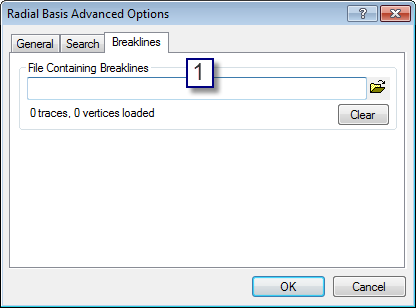The Breaklines section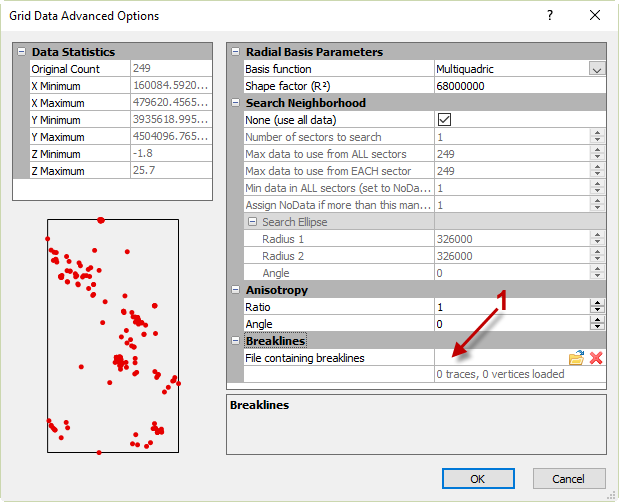is used to upload a blanking file with the breaklines information.
1. A breakline is an interruption in a surface’s slope that is often wavy and irregular, such as the base of a cliff. These are useful to represent any irregularities and disconformities in the map. Breaklines are not barriers when gridding; this means that all data points on both sides of the line are used when interpolating the grid nodes, regardless of the position on either side of the line. However, if a grid node is located on the boundary line then the value of the grid node becomes the value of the boundary file.

The breakline file contains the X, Y, and Z vertices of the interruption in a blanking file (*.BLN) format: the first row is the number of data points in the file followed by the XYZ coordinates of the line.

### Triangulation with Linear Interpolation

The Triangulation with Linear Interpolation works best with data that is evenly distributed over the grid area. If the map is produced with triangular facets then the data set may be too small or most likely contains sparse areas. The gridding method draws lines between data points to create triangles, none of the triangle edges are intersected by other triangles. This gridding method is fast and does not extrapolate beyond the Z value of the data range. In addition, Triangulation with Linear Interpolation does not create data that is outside the data limits; it assigns the NoData value to the grid nodes that are outside the data limits.

The example below shows a data set that was gridded using the Triangulation with Linear Interpolation gridding method.The classed post map, left, was created with the data points. The contour map, left, represents the interpolated grid nodes using the Triangulation gridding method.

To specify the advanced options of the Triangulation with Linear Interpolation gridding method, in the Grid Data dialog, click Advanced Options.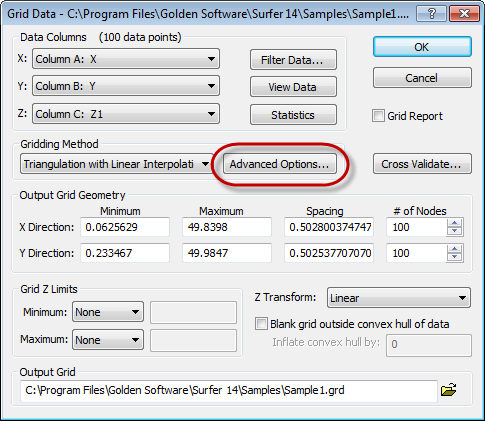Click the Advanced Options button to open theGrid Data Advanced Options dialog.

Anisotropy is useful in eliminating trends in the data when interpolating the grid nodes. Anisotropy refers to directionally dependent data. The Grid Data Advanced Options dialog allows you to specify three different customizations of the gridding. The customizable areas: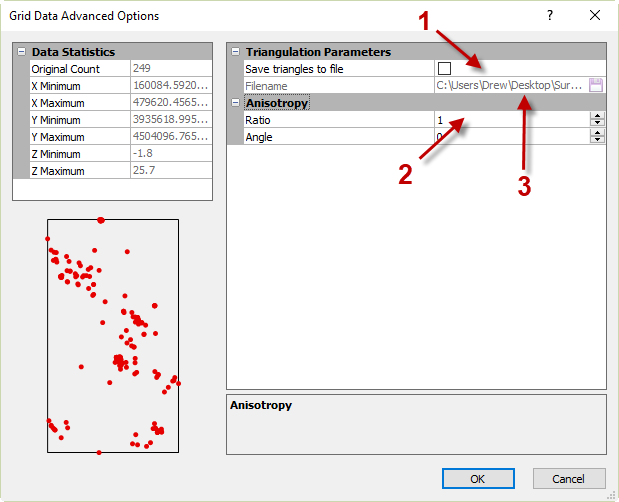The Grid Data Advanced Options allows the anisotropy settings to be specified when gridding the data.
1. Anisotropy Ratio: This value defines the relative weight of the points when interpolating the grid nodes. This value is computed by dividing the maximum range by the minimum range. For most data sets, it is recommended to accept the default value of 1, which means that all points have the same weight. You may want to increase the ratio of the points in one direction that has more similarity than the points in another direction. By increasing the ratio, the points in the direction defined will have more weight on the value of the interpolated grid node. The ratio is typically considered severe if greater than four and mild if less than two.
2. Anisotropy Angle: The angle is the direction of the major axis, in degrees. The angle rotates counterclockwise with 0 degrees being east-west and 90 degrees being north-south going multiple directions. In order to use a direction angle, an anisotropy angle should not equal 1.
3. Save Triangles To: The Delaunay triangles are formed by connecting lines between data points. Often the triangles are formed by maximizing the minimum angle; this prevents small triangles from being formed. The result is triangular faces all over the grid. If two or points are located on the same line then there is no Delaunay triangulation. The triangles that are formed are not intersected by other triangles. The triangles can be useful to determine the density of the data points. The Delaunay triangles can be loaded as a base map and combined with other maps. To save the file, click the folder icon and specify the location and file format to save the file.